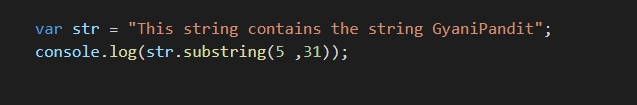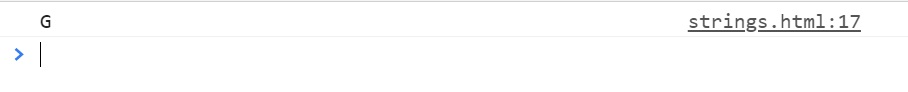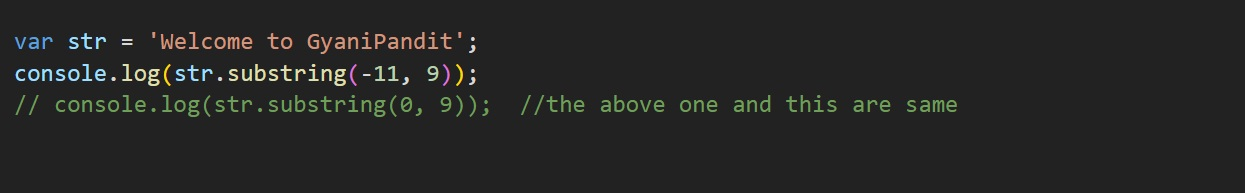# Javascript String Substring Method

Javascript String Substring Method

As the name of the method says it all, this method is going to give us the substring from the given string. Basically, we can understand the substring as just some string inside another string. So, let’s study this method, and we would also have a look at some program, which gives us some idea about this substring method.

A question, for now, should be, what are the things required by the substring method? Well, this method requires two things, the starting position, and the ending position. By the way, the ending position is optional, which means that it is not mandatory to give the ending position argument. If this method is provided with no ending position, it will consider the end, as the end of the string.

So, simply we can say, that the substring method here, is going to return a substring, from the specified start position to the end position(the end position is not included), and if the end position is not provided, then the end is considered as the end of the string.

Lets now try an example, so that we can get the idea of the method substring –As you can see in the program, there is a string, and then we are calling the substring method on that string, with the start and end position values. Now, the substring method is going to return the substring from the start position to the end position(the end position is not included). Let’s have a look at the output now, and we get the substring –As you can see, we got the appropriate substring. But, the thing is that it appears that the slice method and the substring method are doing similar things? But this is not the case every time.

For example, in the slice method, if we are providing the negative arguments, we are getting some string there. But here, in the substring method, if either or both the arguments are negative, or NaN, the substring method treats them as 0. But when it comes to the slice method, if we are giving the negative numbers as arguments, it comes back from the end of the string.

So, you can try having the negative values there. Let’s say if the starting position is given as negative, and the another is positive, then we are going to get the string. Have a look at the below example for this –As you can see, the starting position is provided as negative, so it would be considered as if it is zero, and then the string would be given from 0 to 9. This is pretty much easy to understand. But if you are providing both the arguments as negative, then it is not going to work, and we are going to get the empty string. You can try this thing, and practice some examples so that you can get the idea behind the substring method.